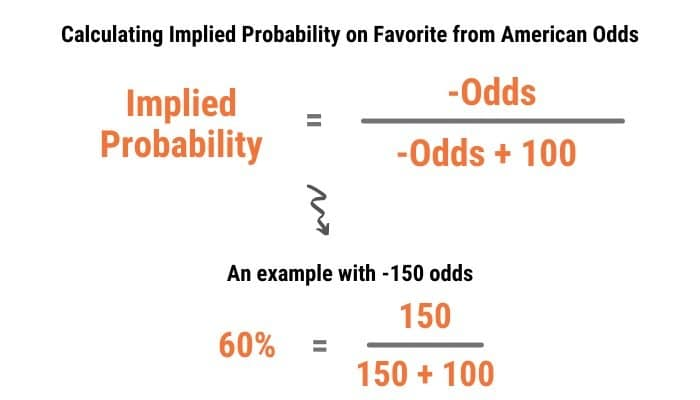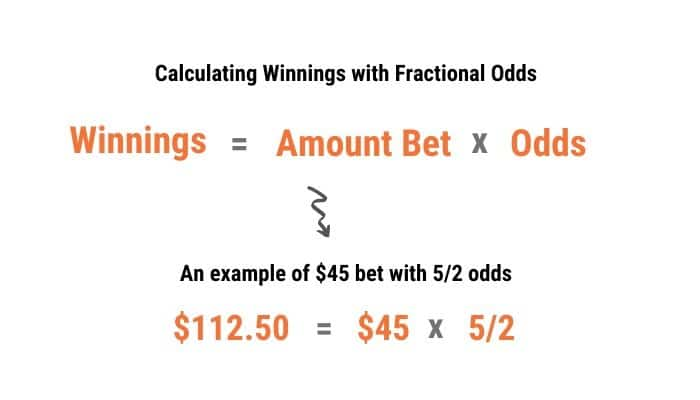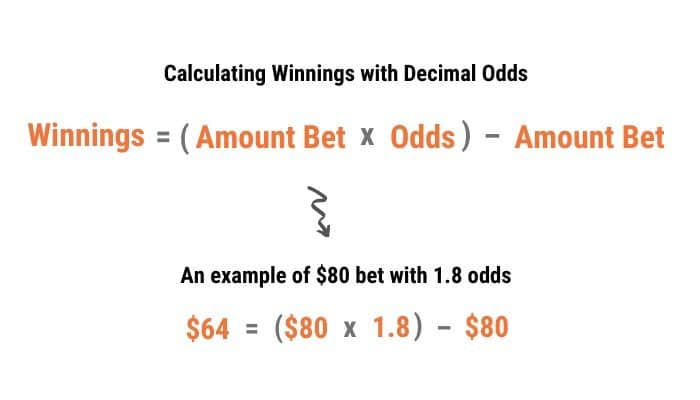# How to Read Betting Odds

The first step to becoming a successful sports bettor is to learn the different types of sports bets and how to read odds.

While reading odds is pretty straightforward, there are 3 different ways odds are typically displayed, most often based on where you live.

Before we dive into the specifics of each of these 3 ways odds are displayed—American, Fractional, and Decimal—let’s take a quick look at a summary of each.

Type

Where Used

\$100 to Win \$150

\$150 to win \$100

American

America

+150

-150

Fractional

U.K. & Ireland

3/2

2/3

Decimal

Europe & Horse Racing

2.5

1.5

One important thing to note in the table above is that American and Fractional odds show the amount you would win (not including the money you staked) while Decimal odds show the total payout (including the money you staked).

If you want to calculate your profit, payout, or implied probability or convert between odds formats, you can quickly do so with our Odds to Probability CalculatorBetting Payout Calculator and Odds Conversion Calculator.

## Why Understanding How to Read Odds is Important

Aside from understanding how much you can win by reading odds, you can also calculate the implied probability of an outcome based on them.

Implied probability is simply the percentage chance that an outcome will occur according to the sportsbook’s odds.

By comparing your perceived chance of an event occurring (such as the Eagles winning) to the implied probability based on the odds, you can find value in betting lines.

It’s important to note that the sum of the implied probabilities for a game will almost always add up to more than 100%. This is because sportsbooks always include “juice” or “vig” into the lines so they can make money.

While there are ways to remove the vig from betting lines, this guide will simply go over how to read odds and convert them to implied probability.

## How to Read American Odds

If you live in the United States, you are most likely to see odds displayed in the American format. These odds will always have a positive (+) or negative (–) sign in front of a number.

### What the + and – mean in sports betting

Many people first see betting lines and ask themselves what the + and – signs mean in sports betting.

Everything with American odds is based on betting or winning \$100, depending on the sign in front of the number.

• For underdogs, there will be a positive sign in front of the number. The number shown reflects how much you would win from a \$100 bet.
• For favorites, there will be a negative sign in front of the number. The number shown reflects how much you would have to bet to win \$100.

For example, if you see the Lakers +140, this means you would win \$140 if you bet \$100 on the Lakers. This does not include the amount you wagered, so if your bet wins, you would collect a total of \$240 (\$140 in winnings + \$100 wagered).

As another example, if you see the Nets -180, this means you would have to bet \$180 to win \$100. This also doesn’t include the amount you wagered, so if your bet wins, you would collect a total of \$280 (\$100 in winnings + \$180 wagered).

### Calculating your winnings in American odds

If you aren’t betting \$100 on every underdog or betting to win \$100 on favorites, you’ll have to do some easy math to calculate your potential winnings with American odds.

If you’re betting on an underdog (+ sign), you simply have to multiply the amount you wagered by the odds divided by 100 to calculate your winnings. Here’s an image that shows the math:If you’re betting on a favorite (– sign), just divide the amount you bet by the odds divided by -100. Here’s an image that shows the math:Quick tip: To divide by 100, simply move the decimal place in the odds two places to the left. For example, you can quickly switch +140 to 1.4. After you do this, you can easily multiply this number by the amount you bet for underdogs or divide the amount you bet by this number for favorites.

### Calculating implied probability from American odds

Like calculating your winnings from American odds, how you calculate implied probability will depend on if you are betting on the underdog or favorite.

To convert American odds to implied probability for underdogs, you must divide 100 by the sum of the odds and 100. For example:To convert American odds to implied probability for favorites, you have to divide the absolute value of the odds (just the odds except positive) by the sum of the absolute value of the odds and 100. For example:#### Why you have to win 52.38% of the time to break even on standard -110 bets

Based on the above equation for calculating implied probability of favorites, you can easily calculate what percentage of bets you have to win to break even at standard -110 lines.Though it may not seem like much, this extra 2.38% is what makes winning in sports betting over the long term so hard.

This is mostly because bookmakers (aka “Vegas”) are very good at setting lines on games. They have a wealth of data, tools, and information that most sports bettors don’t have access to.

## How to Read Fractional Odds

Fractional odds, or British odds, are most common in the United Kingdom, Ireland, and in horseracing.

Any Fractional odds over 1 is an underdog while any Fractional odds under 1 is a favorite.

For example:

• 10/1 odds would be winning \$10 for every \$1 you bet
• 1/10 odds would be winning \$1 for every \$10 you bet

### Calculating your winnings in Fractional odds

Calculating your winning from Fractional odds is as simple as multiplying the amount you wagered by the fraction.

Here is an image showing the math:### Calculating implied probability from Fractional odds

To calculate implied probability from Fractional odds, you just have to divide the denominator of the odds by the sum of the denominator and numerator.

Here is an image showing the math:## How to Read Decimal Odds

Decimal odds are used mostly in parts of Europe (other than the U.K. and Ireland), Australia, New Zealand, and Canada.

As mentioned above, Decimal odds are relative to how much you collect not just how much you win like American and Fractional odds do.

Teams with a 2.0 or over in Decimal odds are underdogs while those under 2.0 are favorites.

### Calculating your winnings in Decimal odds

To calculate your winnings in Decimal odds, you have to multiply the amount you bet by the odds, then subtract the amount you bet from that.

To calculate your total payout in Decimal odds, you just have to multiply the amount you bet by the odds.

Here is an image showing how to calculate winnings from Decimal odds:### Calculating implied probability from Decimal odds

Calculating implied probability from Decimal odds is easier than both American and Fractional odds.

To do so, simply divide 1 by the Decimal odds, like this:## The Importance of Shopping Around for the Best Odds

You may hear people suggest “shopping around” for the best odds before placing your bets.

This is always a good idea.

Since sportsbooks get their lines from various sources (including sometimes making their own), it’s common to find slight differences in the odds offered on the same game.

By comparing the lines at multiple sportsbooks, you can ensure that you are getting the best odds available at the time.

For example, say you want to bet on the Yankees to win straight up (“moneyline”). FanDuel may have odds of -150, DraftKings may have odds of -155, and William Hill may have odds of -165.

In this case, the best book to place your wager with is FanDuel since you will have the highest return if it hits.

When comparing American odds, you want lower negative numbers (as in the example above) and higher positive numbers (since a higher positive number equals are larger payout).

It’s especially important to compare odds on prop and futures bets as these tend to vary much more from sportsbook to sportsbook.

## How Parlay Odds Work

When you place a parlay bet, you get much better odds than you would by simply adding the winnings of each individual bet together.

This is because you need to win each leg of the parlay to win the entire bet.

The following table shows the standard payouts on parlays in which each leg has -110 odds as well as the true probability and fair odds if there were no “juice” or “vig” charged by the sportsbook.

You can also use our Parlay Calculator to quickly calculate your payout and profit based on the odds of each parlay leg.

# Parlay Legs

Implied Probability

Odds

True Probability

Fair Odds

2

27.44%

+264

25%

+300

3

14.37%

+596

12.50%

+700

4

7.53%

+1228

6.25%

+1500

5

3.94%

+2436

3.13%

+3100

6

2.07%

+4741

1.56%

+6300

7

1.08%

+9142

0.78%

+12700

8

0.57%

+17545

0.39%

+25500

9

0.30%

+33585

0.20%

+51100

10

0.16%

+64208

0.10%

+102300

### Dave Rathmanner

Dave Rathmanner is the Founder & CEO of Odds Assist. After struggling to find helpful sports betting resources and honest reviews when he first started betting, he decided to create the site he always wished he had. Dave has been betting on sports since NJ legalized it in 2018 and regularly analyzes sportsbooks to find the best options for bettors. Aside from creating new content for OA readers, Dave is also passionate about researching betting markets, creating models, and developing profitable betting strategies.